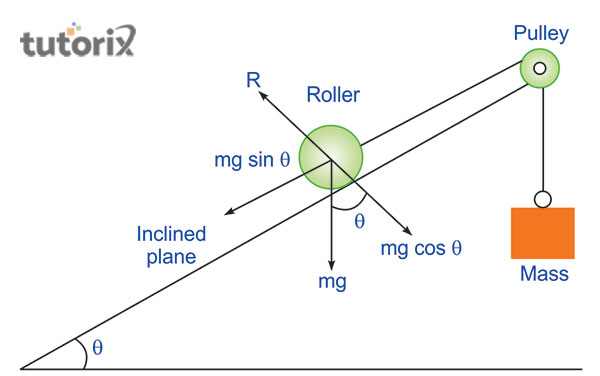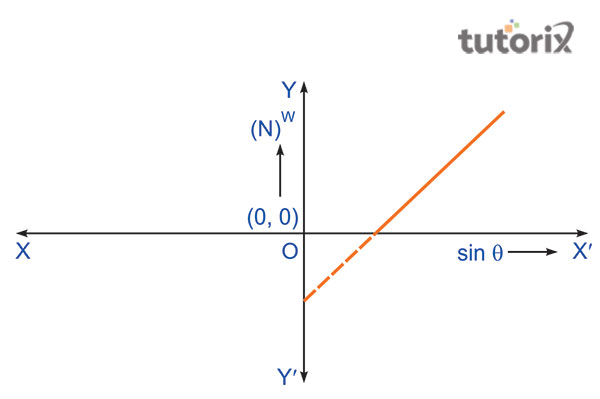# To find downward force along inclined plane on a roller due to gravitational pull of the earth and its relationship with the angle of inclination

The inclination of a certain material proportionally depends on the downward force that is influenced by the gravitational pull of the earth. In this scenario, finding the relationship between these two aspects can depend on a plotting graph in order to outline the relationship with a logical explanation. A particular graph needs to be plotted in order to study the relationship between the angle of inclination (θ) and the downward force denoted by sin θ.

Required materials

The process of finding the relationship involves a series of steps and materials that can help in the succession of the procedure in the first place. Initially, an inclined plane is required that has a protractor and pulley attached to the plane.

After that, a white box and a roller is needed to start the procedure properly. Imminently after taking these materials, the spirit level needs to be balanced for plotting the graph properly.

In addition, a spring balance is required to run the test and find out the relation between the forces.

Finally, a thread and a pan is needed in order to successfully find the relationship between the angle of inclination and the downward force pulled by the gravitational force of Earth.

## DiagramFigure 1: Finding the relation between the angle of inclination and downward force

## Principles

The principle for finding the relationship between the angle of inclination and the downward force needs to include a particular mass, a horizontal angle with the value of θ and a pulley force directed towards the downward direction. Here, the formula will be W= M1h sin θ - fr.Figure 2: Plotting graph of the downward force

In this formula, the M is the mass, and the fr is the friction force caused by the rolling action. In addition, the M1 here is the mass of the roller and the is the total tension of the weight that is suspended.

## Procedure followed

The process of finding the relationship follows an extensive procedure starting from the arrangements of the plane to the repetition of multiple steps in the end.

• The first procedure is arranging the inclined plane, a roller and a particular mass that has a frictionless pulley.
• Then the lubrication process needs to be started if any machines are being used.
• After that, the rollers needs to be suspended and the top plane needs to be adjusted for making the downward direction more sloppy for testing the gravitational pull of the earth (Dou et al. 2021).
• Then, the mass decreasing process needs to be started to create the angle of inclination where the roller will start to fall in the downward direction.
• Finally, the second and the third step needs to be repeated in order to document the observation for future studies.

## Required precautions

In this process, some precautions need to take like the pulley should have to be frictionless. The base of the experiment should have to be horizontal and stable (Learncbse, 2022). The inclined surface should have to be dry, clean and smooth. Here the weights should have to be decreased or increased step by step. The weights in this field are only noted when the roller starts moving up or down.

## Observation table

S. No θ0 Sinθ Mass added to the pan M3 Force W = (M2 + M3)g N
1.
2.
3.

Table 1: Table of Inclination

## Possible sources of error

In this part, it is seen that there can be some possible sources of error in the examination. The pulley here may not be friction-less and the spring balance here may not be accurate (Chauhan et al. 2020). Another source of error in this part can say that the protractor graduations may not be correct. These are the possible sources of error in this part and it speaks many factors of the angle of inclination.

## Conclusion

In this part, an examination is done based on rules of inclination. Here it is said that an inclined plane is the most important aspect of this field. Here the main idea is to find the downward force and it is done along with an inclined plane on a roller. It is done due to the force of the gravitational pull of the Earth. In this part, a relationship is shown with the angle of inclination. It is the main aspect of the tutorial and it speaks about many factors regarding the inclination angle and its relationship with the gravitational force. An inclined plane is the most important aspect here and it is applied for making easy the work process.

## FAQs

Q1. What does it mean by an inclined plane?

An inclined plane is nothing but a wooden plane and it is made smooth with the application of a glass sheet over it. It helps to create an angle with the horizontal ground.

Q2. Is there any relation between the downward force and angle of inclination of the plane?

Yes, there is a relation between these two matters. The relation says that W-mg sin θ is the component of the weight of the body down the plane that is inclined.

Q3. What are the uses of the inclined plane?

There are some uses of the inclined plane and it is nothing but a simple machine that helps to make work easier. It is used on ladders, and on the ramp of the house. It is seen that a plank is put at the back of a truck or it is also used on a railway wagon for unloading and loading heavy goods.

Q4. What are the normal reactions on the inclined plane?

There is a normal reaction of the inclined plane and it says that the reaction is mg cos θ. These are the concepts of the inclined plane and its reaction with the angle.